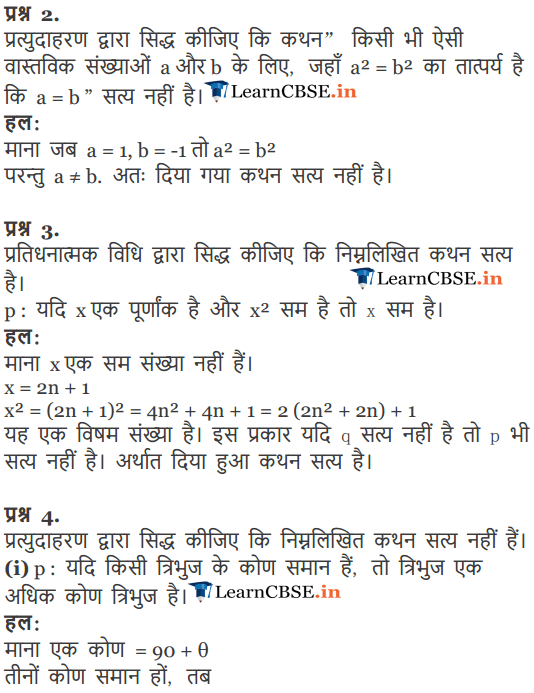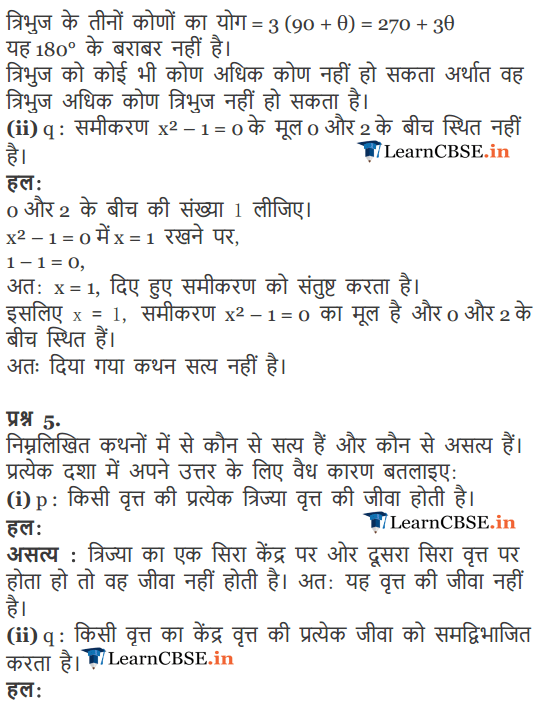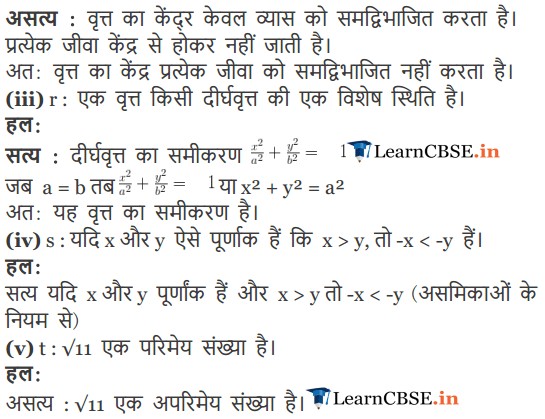Get Free NCERT Solutions for Class 11 Maths Chapter 14 Mathematical Reasoning Ex 14.5 PDF in Hindi and English Medium. Mathematical Reasoning Class 11 Maths NCERT Solutions are extremely helpful while doing your homework. Mathematical Reasoning Ex 14.5 Class 11 Maths NCERT Solutions were prepared by Experienced LearnCBSE.in Teachers. Detailed answers of all the questions in Chapter 14 Class 11 Mathematical Reasoning Ex 14.5 provided in NCERT Textbook.

Free download NCERT Solutions for Class 11 Maths Chapter 14 Mathematical Reasoning Ex 14.5 PDF in Hindi Medium as well as in English Medium for CBSE, Uttarakhand, Bihar, MP Board, Gujarat Board, BIE, Intermediate and UP Board students, who are using NCERT Books based on updated CBSE Syllabus for the session 2019-20.

## NCERT Solutions for Class 11 Maths Chapter 14 Ex 14.5

### NCERT Solutions for Class 11 Maths Chapter 14 Exercise.14.5

Question 1.
Show that the statement
p: “If x is a real number such that x3 + 4x = 0, then x is 0″ is true by
(i) direct method,
(iii) method of contrapositive.
Solution:
The given compound statement is of the form “if p then q”
p: x ϵ R such that x3 + 4x = 0
q: x = 0
(i) Direct method:
We assume that p is true, then
x ϵ R such that x3 + 4x = 0
⇒ x ϵ R such that x(x2 + 4) = 0
⇒ x ϵ R such that x = 0 or x2 + 4 = 0
⇒ x = 0 => q is true.
So, when p is true, q is true.
Thus, the given compound statement is true.

We assume that p is true and q is false, then
x ϵ R such that x3 + 4x = 0
⇒ x ϵ R such that x(x2 + 4) = 0
⇒ x ϵ R such that x = 0 or x2 + 4 = 0
⇒ x = 0.
which is a contradiction. So, our assumption that x ≠ 0 is false. Thus, the given compound statement is true.

(iii) Method of contrapositive: We assume that q is false, then x ≠ 0
x ϵ R such that x3 + 4x = 0
⇒ x ϵ R such that x = 0 or x2 + 4 = 0
∴ statement q is false, so x ≠ 0. So, we have,
x ϵ R such that x2 = -2
Which is not true for any x ϵ R.
⇒ p is false
So, when q is false, p is false.
Thus, the given compound statement is true.

Question 2.
Show that the statement” For any real numbers a and b, a2 = b2 implies that a = b” is not true by giving a counter-example.
Solution:
The given compound statement is of the form “if p then q”
We assume that p is true, then a, b ⍷ R such that a2 = b2
Let us take a = -3 and b = 3
Now, a2 = b2, but a ≠ b
So, when p is true, q is false.
Thus, the given compound statement is not true.

Question 3.
Show that the following statement is true by the method of contrapositive.
p: If x is an integer and x2 is even, then x is also even.
Solution:
The given compound statement is of the form “if p then q”
p: x ϵ Z and x2 is even.
q: x is an even integer.
We assume that q is false, then x is not an even integer.
⇒ x is an odd integer.
⇒ x2 is an odd integer.
⇒ p is false
So, when q is false, p is false.
Thus, the given compound statement is true.

Question 4.
By giving a counter example, show that the following statements are not true.
(i) p: If all the angles of a triangle are equal, then the triangle is an obtuse angled triangle.
(ii) q: The equation x2 – 1 = 0 does not have a root lying between 0 and 2.
Solution:
(i) Since the triangle is an obtuse angled triangle then 0 > 90°.
Let 0 = 100°
Also, all the angles of the triangle are equal.
∴ Sum of all angles of the triangle is 300°, which is not possible.
Thus, the given compound statement is not true,

(ii) We see that x = 1 is a root of the equation x2 – 1 = 0, which lies between 0 and 2. Thus, the given compound statement is not true.

Question 5.
Which of the following statements are true and which are false? In each case give a valid reason for saying so.
(i) p. Each radius of a circle is a chord of the circle.
(ii) q: The center of a circle bisects each chord of the circle.
(iii) r. Circle is a particular case of an ellipse.
(iv) s: If x and y are integers such that x > y, then -x < -y.
(v) t. $$\sqrt { 11 }$$ is a rational number.
Solution:
(i) A chord of a circle is a line whose two endpoints lie on the circle and all the points on the line lie inside the circle. So, the radius of a circle is not a chord of the circle.Thus, the given statement is false.
(ii) The center of a circle bisects chord of circle when the chord is diameter of circle. When the chord is other than diameter then center of circle does not lie on the chord. Thus, the given statement is false.
(iii) In the equation of an ellipse if we put a = b, then we get an equation of circle.
Thus, the given statement is true.
(iv) It is given that x, y ϵ Z such that x > y. Multiplying both sides by negative sign, we have
x, y ϵ Z such that -x < -y.
Thus, the given statement is true.
(v) Since $$\sqrt { 11 }$$ cannot be expressed in the form $$\frac { a }{ b }$$, where a and b are integers and b ≠ 0. Thus, the given statement is false.

### NCERT Solutions for Class 11 Maths Chapter 14 Mathematical Reasoning (गणितीय विवेचन) Hindi Medium Ex 14.5